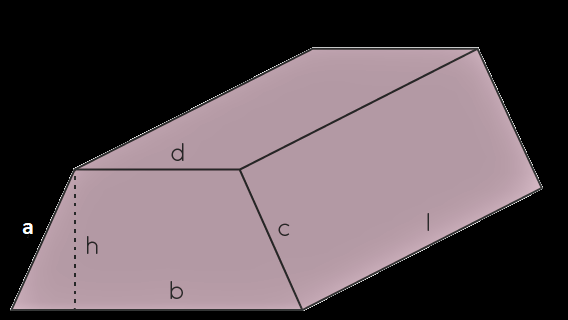1-646-564-2231

# How to find the Area of Trapezoids? – Formula, Examples, DerivationWhat is a trapezoid? A trapezoid or trapezium is a quadrilateral with at least one pair of parallel sides. The parallel sides are known as bases. When the other two sides are non-parallel, they are called legs or lateral sides. Else there are two pairs of bases. Real-life examples where you can see the area of trapezoids are handbags, popcorn tins, and the guitar-like dulcimer. The area of a trapezoid is the complete space enclosed by its four sides. There are two approaches to finding the area of trapezoids.

1. The first method is a direct method that uses a direct formula to find the area of a trapezoid with the known dimensions (see example 1)
2. For the second method, firstly, if we are given the length of all the sides, we split the trapezoid into smaller polygons such as triangles and rectangles. Next, we will find the area of the triangles and rectangles separately. Finally, we will add the area of the polygons to get the total area of the trapezoid. (see example 2 for  a more precise understanding)

## What is the Formula To Calculate the Area of Trapezoids?

We can calculate the area of a trapezoid if we know the length of its parallel sides and the distance (height) between the parallel sides. The area of trapezoids formula is:

A = ½ (a + b) h

Here (A) is the area of a trapezoid.

‘a’ and ‘b’ are the parallel sides of the trapezoid

‘h’ is the height, i.e., the perpendicular distance between the parallel sides.

## Area of Trapezoid Example

Here is an area of a trapezoid example using the direct formula and an area of a trapezoid example with the alternative method.

## Trapezoidal Prism

A trapezoidal prism is a 3D figure made up of two congruent trapezoids that are connected by four rectangles. The trapezoids are on the top and the bottom. Thus, they form the base for prisms and have polygons that form their bases. The four rectangles form the lateral faces of the trapezoid prism. So, a trapezoidal prism consists of-

• Six faces
• Eight vertices
• Twelve edges

### Surface Area of a Trapezoidal Prism

The area of a trapezoidal prism is the sum of the surfaces of the prism. This area equals the areas of all of the faces of the trapezoid prism. Since a trapezoidal prism has two trapezoidal faces and four rectangular faces, a sum of their areas will give the surface area of the prism. However, there is a simple and direct formula for calculating the surface area of a trapezoid prism. The formula is:

Surface Area of a Trapezoidal Prism = h (b + d) + l (a + b + c + d) unit square.

Here, h = height

b and d are the lengths of the base

a + b + c + d is the perimeter

l is the lateral surface area of a trapezoidal prism

## Derivation of Surface Area of a Trapezoidal PrismThe base of a trapezoidal prism is trapezoid in shape. Here,

b and d are parallel sides of the trapezoid

H = distance between the parallel sides

l = length of the trapezoidal prism

So, the total surface area of a trapezoidal prism (TSA) = 2 × areas of base + lateral surface area ————- (1)

Area of a trapezoid =  ½ (base 1 + base 2) height

So, area of trapezoidal base = h (b + d)/2 ——————— (2)

The lateral area of a trapezoidal prism (LSA) = sum of the areas of each rectangular surface

So, LSA = (a × l) + (b × l) + (c × l) + (d × l) ——————- (3)

On substituting the values from equation (2) and equation (3) in equation 1 i.e., TSA formula, we get:

(TSA) = 2 × h (b + d)/2 + (a × l) + (b × l) + (c × l) + (d × l)

TSA = h (b + d) + [(a × l) + (b × l) + (c × l) + (d × l)]

Total surface area of a trapezoidal prism = h (b + d) + l (a + b + c + d)

Thus, TSA of a trapezoidal prism = h (b + d) + l ( a + b + c + d) unit square

How to Find the Surface Area of a Trapezoidal Prism?

Here are the steps on how to find the surface area of a trapezoidal prism.

Step 1: Spot the four sides of the trapezium – a, b, c, and d. These represent the widths of the four rectangles. The addition of these 4 values will give the perimeter P.

Step 2: Spot the length h of the prism.

Step 3: Find the lateral area of a trapezoidal prism.

Step 4: Identify b1, b2 and h of the trapezoid. Now, find the base area B using the formula, (b1 + b2) h/2

Step 5: Lastly, put the values in the formula = 2B + Lateral Surface Area to calculate the total surface area of the trapezoidal prism.

Consider the following example of the surface area of a trapezoidal prism for a clearer understanding.

### TESTIMONIALS## More Related TOPICS

### Use Models to Subtract Mixed Numbers

Key Concepts Using models to subtract mixed numbers Subtract mixed numbers Addition and subtraction of mixed

### Use Models to Add Mixed Numbers

Key Concepts Using models to add mixed numbers Adding mixed numbers Introduction  In this chapter, we

### Find Common Denominators

Key Concepts Equivalent fractions and common denominators Finding common denominators Introduction In this chapter, we will